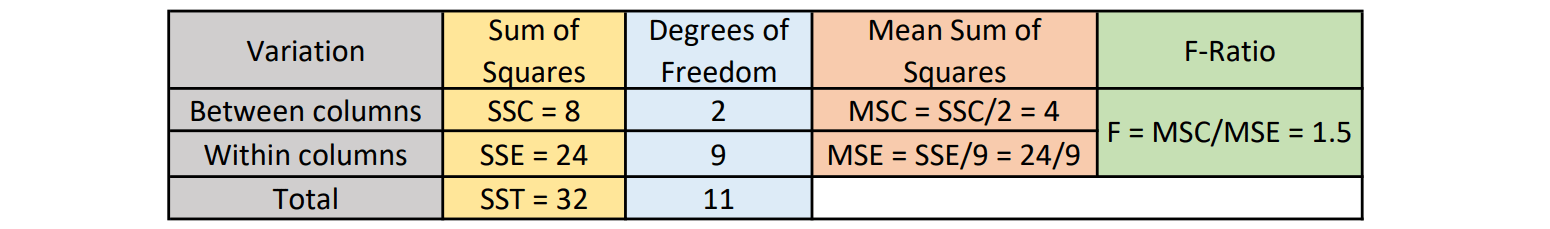### Introduction

Analysis of Variance or ANOVA is a statistical procedure of inference of the acceptability of the null hypothesis when three or more population means are involved. ANOVA is a tool that splits the observed aggregate variability into systematic factors and random factors.

ANOVA was developed by the famous statistician Ronald Fisher, and it is based on the Law of Total Variance. It uses the F-Test method to conclude the significant difference in the means of the population.

### One-Way ANOVA

The one-way ANOVA determines the hypothesis acceptability of a single factor or variable only among the given number of populations. We use one-way ANOVA when we want to separately prove the significant difference of each factor or the main factor.

One-way ANOVA follows a Completely Randomized Design where the treatment of the experimental units is random. The total variation is divided into two components, Treatment and Error.

### Algorithm

The following are the steps to use one-way ANOVA to test the significant difference among various populations:

Step 0: Initialize the null hypothesis and the alternative hypothesis.

Step 1: Find the total number of observations, N.

Step 2: Calculate the total of all observations, T.

Step 3: Find the correction factor, T^2/N.

Step 4: Calculate the total sum of squares, SST.

Step 5: Calculate the column sum of squares, SSC.

Step 6: Calculate the error sum of squares, SSE = SST – SSC.

Step 7: Find the degrees of freedom between columns and for the error.

Step 8: Calculate the mean sum of squares MSC (for column) and MSE (for error).

Step 9: Calculate the F-ratio and compare it with the table value of F-test to infer whether the null hypothesis can be accepted or rejected.

Note: In steps 4 and 5, we must subtract the sum of squares with the correction factor.

### Implementation

Let us consider an example where various varieties of wheat are grown on various plots of land for a given duration. The values are given below:We can try to infer whether there is a significant difference between the 3 varieties of wheat using one-way ANOVA by following the algorithm mentioned above:

We can initialize our null hypothesis to conclude the fact that the mean of all the wheat varieties is equal. The alternative hypothesis will conclude otherwise.The following table calculates the sum of elements as well as the sum of squares:Step 1: N = No. of rows(R) x No. of columns(C), i.e., 4 x 3 = 12.

Step 2: T = 24 + 20 + 16 = 60.

Step 3: Correction factor = 3600/12 = 300.

Step 4: SST = 158 + 108 + 66 – 300 = 32.

Step 5: SSC = (24)^2/4 + (20)^2/4 +(16)^2/4 – 300 = 8.

Step 6: SSE = 32 – 8 = 24.

Step 7: Degrees of freedom within the column = N – C = 12 – 3 = 9.

Degrees of freedom between the columns = C – 1 = 2.

We can tabulate the answers yielded to easily continue with step 8 and 9.From the table above:

Step 8: MSC = 4 and MSE = 24/9.

Step 9: F-ratio = 1.5.

The table value of df = (9,2) at 5% significance is 4.2565. The F-test table values for various levels of significance can be found here.

Since the F-ratio is less than the table value, we accept the null hypothesis. This means that there is no significant difference between the means of the yields of the variety of wheat crops among the various plots of land.

Let us try to code the example above by creating a function that can return the final F-ratio value.

				
fun one_anova(arr: Array): Double
{
val c = arr.size
val r = arr.size
val n = r * c
var sum = 0.0
var sst = 0.0
var ssc = 0.0
var f = 0.0
val t = DoubleArray(c)
val sqarr = DoubleArray(c)
for (i in 0 until c)
{
for (j in 0 until r)
{
sum += arr[i][j]
t[i] += arr[i][j]
sqarr[i] += arr[i][j] * arr[i][j]
}
}
val cfac = sum * sum / n
for (i in sqarr.indices)
{
sst += sqarr[i]
ssc += t[i] * t[i]
}
sst -= cfac
ssc = ssc / r - cfac
val sse = sst - ssc
val msc = ssc / (c - 1)
val mse = sse / (n - c)
f = if (msc &gt; mse)
{
msc / mse
}
else
{
mse / msc
}
return f
}



The table values can be taken as an input using 2-dimensional arrays wherein each 1-dimensional array represents the values of a population.

Also, the table value of F for the given degrees of freedom can be found using the statistical tables.

				
val arr = arrayOf(
doubleArrayOf(6.0, 7.0, 3.0, 8.0),
doubleArrayOf(5.0, 5.0, 3.0, 7.0),
doubleArrayOf(5.0, 4.0, 3.0, 4.0))
val exp = 4.26
val ans = one_anova(arr)
println("F value: "+ans)
if (ans &gt; exp)
{
println("Significant difference")
}
else
{
println("No significant difference")
}



Output:

				
F value: 1.5
No significant difference